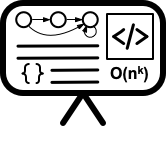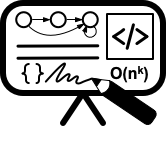# Lecture 1 - Languages and regular expression

Date Pre-lecture slides Post-lecture scribbles Lecture recording
August 24 2023## Notes

#### Strings and Languages

• Strings are the elements of the languages. Each string represents a problem instance.
• A alphabet($\Sigma$) is a finite set of symbols (example: $\Sigma = \{0, 1\}$).

Some string and set facts:

Strings:
• $x \cdot y = xy$ is the concatenation of two strings.
• $\vert w \vert$ is the length of a string
• $\Sigma^n$ is the set of all strings over $\Sigma$ of length $n$
• $\Sigma^{*}$ is the set of all strings over $\Sigma$ of all lengths
• $\varepsilon$ is the empty string
• Subsequence of string is a subset of its characters that appear in the same order as they do in the original string
Sets:
• $\emptyset$ is the empty set
• $\\{ \varepsilon \\}$ is the non-empty set containing one element, the empty string.
• Concatentation of two sets is all possible pairs of elements

#### Terminology

• A character(a,b,c,x) is a unit of information represented by a symbol: letters, digits, whitespace
• A alphabet($\Sigma$)is a set of characters
• A string(w) is a sequence of characters
• A language(A,B,C,L) is a set of strings
• A grammar(G) is a set of rules that defines the strings that belong to a language

#### Regular Languages

Kleene’s Theorem: A language is regular if and only if it can be obtained from finite languages by applying the three operations: union ($\cup$), concatenation ($\cdot$), repetition($^*$) a finite number of times.

Base Case:: $\emptyset$, $\{ \varepsilon \}$, $\{a\}$ (for each $a \in \Sigma$) are all regular languages. Inductive Step: If you can apply the above operations on the base language a

#### Regular expressions

A simple shorthand for describing a regular language. IN regular expressions:

• $\emptyset$ denotes $\emptyset$
• $\varepsilon$ denotes $\{\varepsilon\}$
• $a$ denotes $\{a\}$
• $r_1+r_2$ denotes $R_1 \cup R_2$
• $r_1 \cdot r_2$ denotes $R_1R_2$
• $r^*$ denotes $R^*$

#### Everything tied together

Let’s look at the following problem:

Problem: Consider the problem of a n-input AND function. The input ($x$) is a string $n$-digits long with $\Sigma = {0,1}$ and has an output ($y$) which is the logical AND of all the elements of $x$.

TO analyze it’s computational complexity, we need to formulate it as a language ($\Sigma = \{0, 1, \cdot, \vert \}$):

$L_{AND_N} = \begin{Bmatrix} 0\cdot|0, & 1\cdot|1, & & \\ 0 \cdot 0\cdot| 0, & 0 \cdot 1\cdot| 0, & 1 \cdot 0\cdot| 0, & 1 \cdot 1\cdot| 1 \\ \vdots & \vdots & \vdots & \vdots \\ (0\cdot)^n|0, & (0\cdot)^{n-1}1|0, & \ldots & (1\cdot)^n|1 \ldots \\ \end{Bmatrix}$

Then to show it’s one of the simplest languages there is, we represent that language as a regular expression:

$r_{AND_N} = \underbrace{(0\cdot + 1\cdot)^* 0 (0\cdot + 1 \cdot)^* \vert 0"}_{\text{all output 0 instances}} + \overbrace{( 1 \cdot)^*\vert 1}^{\text{all output 1 instances}}$

#### Things I forgot to mention

###### What is $\varepsilon^+$

There was a question on what $\varepsilon^+$ (and $\varepsilon^*$). My argument was that it should be $\{\varepsilon\}$ because you always get a set out of the Kleene star and:

$\varepsilon^+ = \Sigma_{n=1}^{\infty}\varepsilon^n = \varepsilon^1 \cup \varepsilon^2 \cup \ldots$

where you have multiple strings that you can union together. However, some of you were confused because theres only strings in that equation and so shouldn’t the output be a string ($\varepsilon$ (not a set))?

I went through a bunch of text and saw that Kleene star is always applied to a set. The only time when it isn’t applied to a set is when we’re talking about regular expressions.

So I think you guys are right, sort of. Union is supposed to be a set operation and union-ing strings together means nothing. However, the issue is regular expressions are a permanent fixture of modern computability and so when someone writes $w^*$, they don’t literally mean union of $w$ with $ww$ and so on, in the mathematical sense, they mean $w^*$ in the RegEx sense and regular expressions assume that when you write $w$, you mean $\{w\}$. So yeah, this is my bad.

The correct answer would be $\{\varepsilon\}$ assuming a regular expression, or simply undefined in a strict mathematical interpretation.